# Elimination Method

James went grocery shopping and bought 2 boxes of cereals and 1 carton of milk for $70. The following week, he bought 1 box of cereals and 2 cartons of milk for$75. He wants to know the price per item for the cereal box and milk carton.Can we help James solve his query?

To solve his problem, James needs to apply a method of solving linear equations in two variables. This page is exactly what he is looking for.

In this chapter, we will learn about solving linear equations in two variables using the elimination method.

## Lesson Plan

 1 How Does One Solve a Linear Equation by the Elimination Method? 2 Challenging Questions 3 Solved Examples on Elimination Method 4 Interactive Questions on Elimination Method 5 Thinking Out of the Box!

## Solving Linear Equation by Elimination Method

There are five most commonly used methods to solve the system of equations which are given below:

• Graphical Method
• Elimination Method
• Substitution Method
• Cross Multiplication Method
• Matrix Method

Of these methods, the elimination method is one of the most widely used methods to solve the system of equations.

The elimination method is widely used because in this method we eliminate one of the variables and do our calculation with only one variable quickly.

Let us learn more about this method.

### How to Do the Elimination Method?

• Let us assume two linear equations in the general form:
• Select a variable which you want to eliminate from the equations.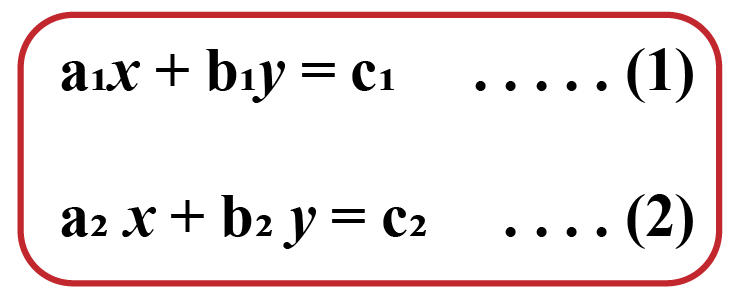Here, we have chosen to eliminate "$$x$$".

• Take suitable constants and multiply them with the given equations so as to make the coefficients of the variable (which we want to eliminate) equal.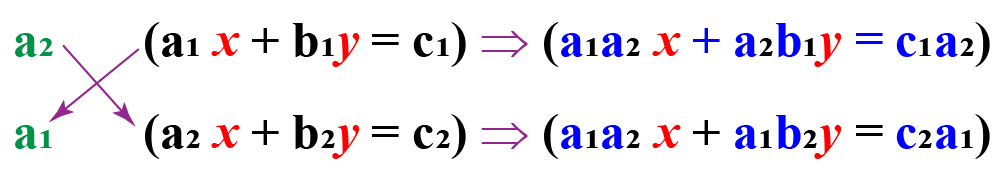Here, the coefficient of x becomes $$a_1 a_2$$.

• Add or subtract the two thus obtained equations and eliminate one variable.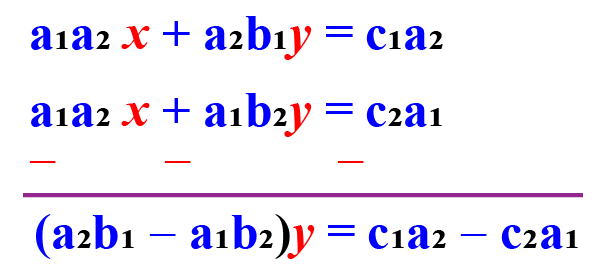Here, we subtract the second equation from the first.

• Solve the equation to find the value of the remaining variable.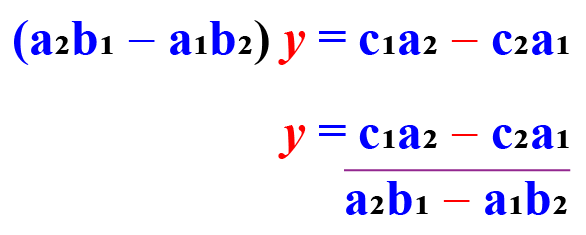• Substitute the value of the variable to find the value of the other variable.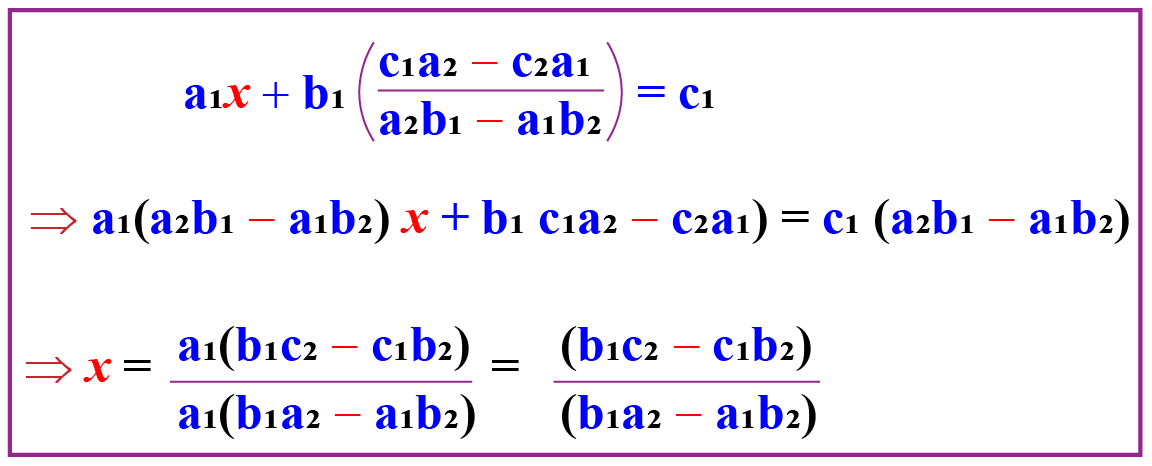Therefore,

 $$x = \dfrac {b_1 c_2 - c_1 b_2}{b_1 a_2 - a_1 b_2}, y = \dfrac{c_1 a_2 - c_2 a_1}{a_2 b_1 - a_1 b_2}$$

Examples

Let us take an example.

Question

Find the value of $$x$$ and $$y$$ from the set of linear equations given below:

\begin{align*} 4x - 3y &= 32 \quad ...(1) \\ x + y &=1 \quad ...(2) \end{align*}

Solution

Step 1: Select a variable which you want to eliminate from the equations.

Let us select $$y$$.

\begin{align*} 4x - 3 \color{blue} {y} &= 32 \\ x + \color{blue} {y} &=1 \end{align*}

Step 2: Take suitable constants and multiply them with the given equations so as to make the coefficients of the variable (which we want to eliminate) equal.

Let us multiply the second equation by 3

\begin{align*} \color{red}3 \times (x + \color{blue} {y} &=1 ) \\ \color{red}3 x \ + \color{red}3 \color{blue} {y} &= \color{red} { 3 } \quad ...(3) \end{align*}

Step 3: Add or subtract the two thus obtained equations and eliminate one variable.

Here, on adding the equations (2), and (3), we get,

\begin{align*} 4x \ - 3 \color{blue} {y} &= \quad 32 \\ \color{red}3 x \ + \color{red}3 \color{blue} {y} &= \color{red} { \ \ \quad 3 } \\ \underline{+\qquad+ \: \quad } &= \underline{ +\quad \ } \\ 7x \qquad \: \: &= \quad 35 \end{align*}

Step 4: Solve the equation to find the value of the remaining variable.

On solving, we get, \begin{align*} 7x &= 35\\ x &= 5 \end{align*}

Step 5: Substitute the value of the variable to find the value of the other variable.

On substituting the value of $$x$$ in equation 1, we get,\begin{align*} 4(5) - 3 \color{blue} {y} &= 32 \\ \color{blue} {y} &= \dfrac{32-20}{-3} \\ \color{blue} {y} &= -4\end{align*}

Therefore the value of x = 5 and y = -4

The Difference Between the Substitution Method And Elimination Methods

The difference between the substitution method and elimination methods are listed in the table below:

Elimination Method Substitution Method
The elimination method consists of eliminating the same variable from two equations and then we find the value of another variable that was not eliminated after taking the difference of two equations. Then the obtained value of one variable is placed in either of the two equations. further, the value of another variable can be found after simplification. The substitution method consists of solving a variable in the function of another variable and then substituting the value of the first variable into another equation, due to which equation becomes the function of a single variable. the value of the variables can be found after simplification.
The elimination method is generally used when the coefficients of variables are not equal to 1. If the coefficient of any variable is 1, in any one of the equation, then it is easier to use the substitution method.

## Elimination Method Calculator

Check out the following substitution method calculator to solve any pair of linear equations in two variables.Challenging Questions
• Solve the equation by substitution method if $$6m-3n=32 \text{ and } x+y=-1$$.

• The sum of the successors of the two numbers is 36 and the difference of their predecessors is 8. What are the numbers?

## Solved Examples

 Example 1

Dorion was given two equations $$5m-2n=17$$ and $$3m+n=8$$ and asked to find the value of $$m$$ and $$n$$. Can you help him in finding the value of $$m$$ and $$n$$ using the elimination method?

Solution

We have,

\begin{align*} 5m-2n &= 17 \qquad ...(1) \\ 3m+n &=8 \qquad ...(2) \end{align*}

The solution of the given two equations can be found by following steps:

• Select a variable which you want to eliminate from the equations.

\begin{align*} 5m-2 \color{blue} {n} &= 17 \\ 3m + \color{blue} {n} &=8 \end{align*}
Here, we have selected "$$\color{blue} {n}$$" to eliminate.

• Take suitable constants and multiply them with the given equations so as to make the coefficients of the variable (which we want to eliminate) equal.
Multiply the second equation by 2.
\begin{align*} 2 \times (3m + \color{blue} {n} &=8) \\ \implies \qquad 6m + 2\color{blue} {n} &= 16 \qquad ...(3) \end{align*}
Here, the coefficient of $$n$$ becomes $$2$$.
• Add or subtract the two thus obtained equations and eliminate one variable.
Here, we add the equations (1) and (3).\begin{align*}5m-2 \color{blue} {n} &= \quad17 \\ 6m + 2\color{blue} {n} &=\quad16 \\ \underline{+\qquad+ \: \quad } &= \underline{ + \qquad\ } \\ 11m \qquad \: \: &= \quad 33 \end{align*}
• Solve the equation to find the value of the remaining variable.
On solving, we get,
\begin{align*} 11m &= 33 \\ m &= \dfrac{33}{11} = 3 \end{align*}
• Substitute the value of the variable to find the value of the other variable.
Substituting the value of n in Eq. (1), we have,

\begin{align*} 5m-2 \color{blue} {n} &= 17 \\ 5(3) -2 \color{blue} {n} &= 17 \\ \color{blue} {n} &= \dfrac {15 - 17}{2} \\ \color{blue} {n} &= -1 \end{align*}

 $$\therefore \\$$ m = 3, and n = -1
 Example 2

Jack has two numbers, the sum of the two numbers is 20 and the difference between them is 10. Can you find the numbers?

Solution

Let the two numbers be x and y.

Given that:

\begin{align*} x+y&=20 \quad ...(1) \\ x-y&=10 \quad ...(2) \end{align*}

Here, coefficient of x is same in both the equations, therefore, on subtracting the (2) equation from (1) equation, we get

\begin{align*}x+y&=\quad20 \\ +x-y&=+\ 10 \\ \underline{-\quad- \ \:} &= \underline{ - \quad\ } \\ \quad 2y &= \quad 10 \\ \implies \qquad y&=\quad \ 5\end{align*}

Substituting the value of y in eq (1), we get,

\begin{align*} x+y&=20 \\ x+5&=20 \\ \implies \qquad x&=15 \end{align*}

 $$\therefore$$    x = 15, and y = 5
 Example 3

Jonathan has a box, the total number of $1 coins and$2 coins is 20 in that box. If the total coins amount to $46, find the number of coins of each denomination.Solution Let the number of$1coins and 2 coins be x and y respectively. Given that: \begin{align*} x+y &=20 \quad ...(1) \\ 2x+5y&=46 \quad ...(2) \end{align*} Consider the first equation and multiply it by 2 \begin{align*} 2 \times (x+y&=20) \\ 2x + 2y &=40 \quad ...(3) \end{align*} On subtracting the (3) equation from (2) equation, we get \begin{align*}2x+5y&=\quad 46 \\ 2x + 2y &=\quad 40 \\ \underline{-\quad- \ \:} &= \underline{ - \quad\ } \\ \quad 3y &= \quad \ 6 \\ \implies \qquad y&=\quad \ 2\end{align*} Substituting the value of y in the first equation, we have, \begin{align*}x+2&=20\\ x&=20-2\\ \implies \qquad x&= 18\end{align*}  $$\therefore$$ Number of1 coins =18   Number of \$2 coins = 2

## Interactive Questions

Here are a few activities for you to practice. Select/Type your answer and click the "Check Answer" button to see the result.Think Tank
1. How many solutions are there for linear equations in two variables?

## Let's Summarize

We hope you enjoyed learning about the elimination method with the interactive questions. Now, you will be able to easily solve problems on the elimination method.

## About Cuemath

At Cuemath, our team of math experts is dedicated to making learning fun for our favorite readers, the students!

Through an interactive and engaging learning-teaching-learning approach, the teachers explore all angles of a topic.

Be it worksheets, online classes, doubt sessions, or any other form of relation, it’s the logical thinking and smart learning approach that we at Cuemath believe in.

## Frequently Asked Questions (FAQs)

### 1. What is the elimination method?

It is a method to solve the system of equations.
In this method, we eliminate one of the two variables and try to solve equations with one variable, and proceed further.

### 2. How do you solve linear equations using the elimination method?

At first, we select a variable which we want to eliminate from the equations.

Then take suitable constants and multiply them with the given equations so as to make the coefficients of the variable (which we want to eliminate) equal.

Add or subtract the two thus obtained equations and eliminate one variable.

Solve the equation to find the value of the remaining variable.

Substitute the value of the variable to find the value of the other variable.

### 3. Write down the steps involved in the elimination method.

Step 1: At first, we select a variable which we want to eliminate from the equations.

Step 2: Take suitable constants and multiply them with the given equations so as to make the coefficients of the variable (which we want to eliminate) equal.

Step 3: Add or subtract the two thus obtained equations and eliminate one variable.

Step 4: Solve the equation to find the value of the remaining variable.

Step 5: Substitute the value of the variable to find the value of the other variable.

### 4. How do you solve two equations using elimination?

At first, we select a variable which we want to eliminate from the equations.

Then take suitable constants and multiply them with the given equations so as to make the coefficients of the variable (which we want to eliminate) equal.

Add or subtract the two thus obtained equations and eliminate one variable.

Solve the equation to find the value of the remaining variable.

Substitute the value of the variable to find the value of the other variable.

### 5. What do you mean by elimination in linear equations?

It is a method to solve the system of equations.
In this method, we eliminate one of the two variables and try to solve equations with one variable, and proceed further.

### 6. Can the elimination method be used to solve the system of equations in three variables?

Yes, the elimination method can be used to solve the system of equations in three variables.

### 7. What is the benefit of the elimination method?

It is a method to solve the system of equations.
In this method, we eliminate one of the two variables and try to solve equations with one variable, and proceed further.

### 8. How do you solve substitution and elimination?

Substitution method:
In the substitution method, the value of any one variable from anyone equation is substituted in the other equation.

Elimination method:
In the elimination method, we either add or subtract the equations to get the solution.

### 9. How many solutions are there for linear equations in two variables?

There are three types of solutions for linear equations in two variables.

1. There are zero solutions. 2. There is one solution. 3. There are infinitely many solutions.

### 10. What is a two-variable equation?

A linear system of equations with two variables is called a two-variable equation.

Download Pair of Linear Equations in 2 Variables Worksheets
Pair of Linear Equations in 2 Variables
grade 10 | Answers Set 1
Pair of Linear Equations in 2 Variables
grade 10 | Questions Set 2
Pair of Linear Equations in 2 Variables
grade 10 | Answers Set 2
Pair of Linear Equations in 2 Variables
grade 10 | Questions Set 1

More Important Topics
Numbers
Algebra
Geometry
Measurement
Money
Data
Trigonometry
Calculus